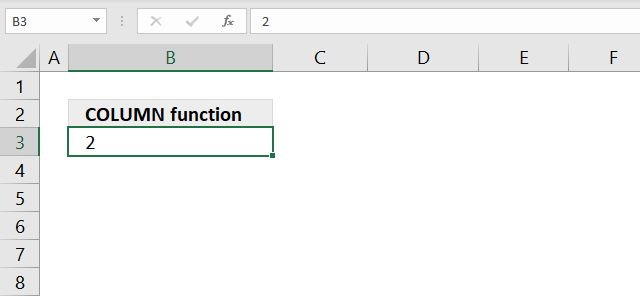Author: Oscar Cronquist Article last updated on March 30, 2021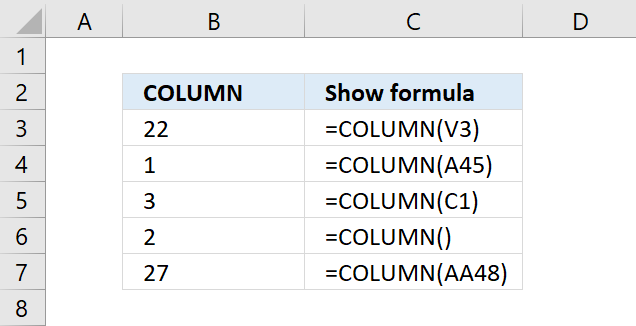The COLUMN function returns the column number of the top-left cell of a cell reference. If the argument is not entered the column number of the cell the formula is entered in is returned, see cell B6 in the picture above.

### Excel Function Syntax

=COLUMN(reference)

### Arguments

 reference Optional. The cell reference for which you want to extract the column number from.

The COLUMN function allows you to also return an array of column numbers if you enter the formula as an array formula and use a cell range containing multiple columns.

You can not use multiple cell references in the COLUMN function.

## 1. How to convert a column number to a column letter?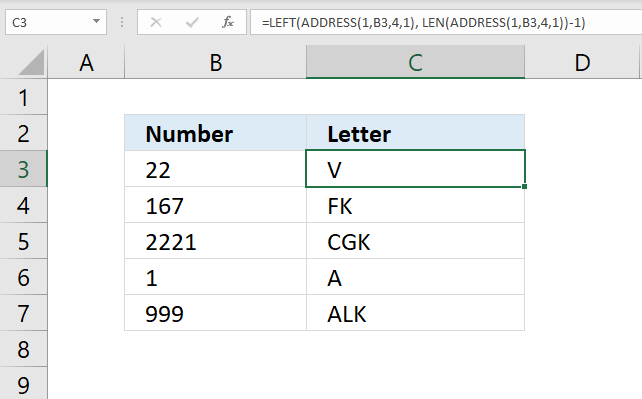The formula in cell C3 calculates the column letter from a column number. Column number 1 is column A, column 2 is B, and so on. See the image above.

Formula in cell C3:

=LEFT(ADDRESS(1, B3, 4, 1), LEN(ADDRESS(1, B3, 4, 1))-1)

### Explaining formula in cell C3

#### Step 1 - Calculate cell address

The ADDRESS function returns the address of a specific cell, you need to provide a row and column number.

ADDRESS(row_numcolumn_num, [abs_num], [a1], [sheet_text])

becomes

and returns "V1".

#### Step 2 - Calculate number of characters in cell address

The LEN function calculates the number of characters in a given string.

becomes

LEN("V1")-1

becomes

2-1 equals 1.

#### Step 3 - Extract column letter from cell address

The LEFT function extracts a specific number of characters always starting from the left.

becomes

LEFT("V1", 1)

and returns "V" in cell C3.

## 2. How to convert a column letter to a column number?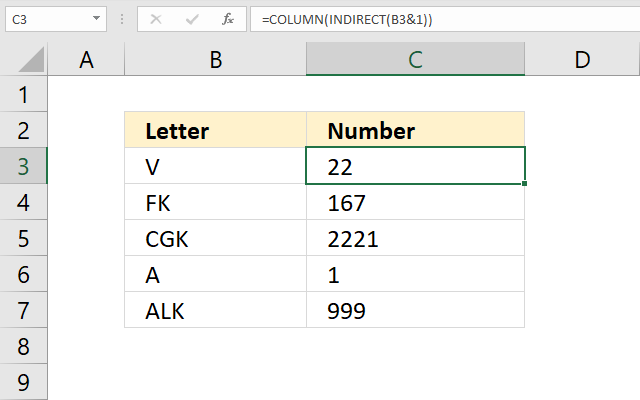The formula in cell C3 calculates the column number from a column letter. Column number 1 is column A, column 2 is B, and so on. See the image above.

Formula in cell C3:

=COLUMN(INDIRECT(B3&1))

### Explaining formula in cell C3

#### Step 1 - Concatenate strings

The ampersand character lets you concatenate values.

B3&1

becomes

"V"&1

and returns "V1".

#### Step 2 - Create cell reference

The INDIRECT function returns the cell reference based on a text string.

INDIRECT(B3&1)

becomes

INDIRECT("V1")

and returns cell reference V1.

#### Step 3 - Calculate column number

COLUMN(INDIRECT(B3&1))

becomes

COLUMN(V1)

and returns 22 in cell C3.

## 3. How can the COLUMN function return an array?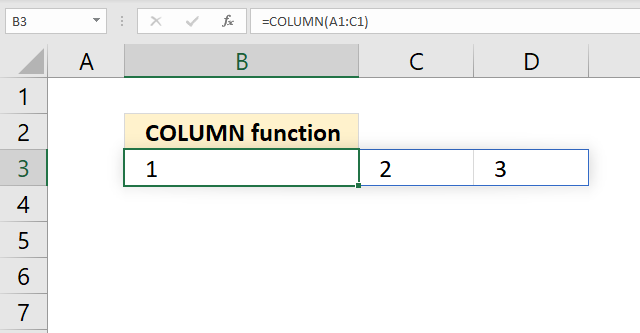The COLUMN function calculates the column number from a cell reference, the column function returns an array of numbers if the cell reference points to a cell range that has multiple columns.

Formula in cell B3:

=COLUMN(A1:C1)

The formula in cell B3 returns a horizontal array of numbers from 1 to 3 representing the column numbers of cells A1, B1, and C1. Column letter A is 1, column 2 is B, and so on.

You need to enter this formula in cell B3 as an array formula if you own an earlier Excel version than Excel 365.

## 4. COLUMN function and VLOOKUP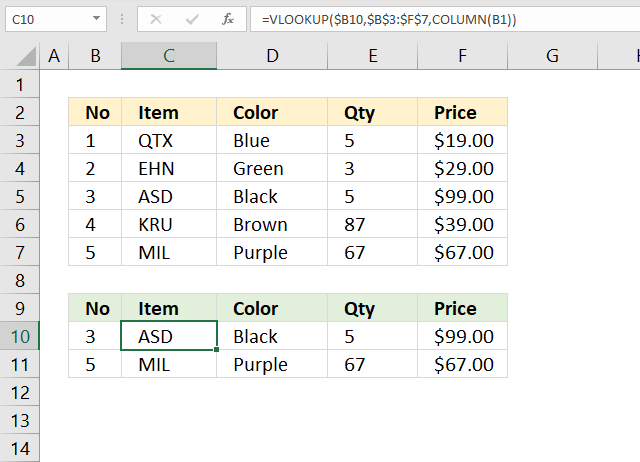The formula in cell C3 combines the VLOOKUP and the COLUMN functions to get all values from the same row if the lookup value matches the leftmost value in the table array.

Formula in cell B3:

=VLOOKUP(\$B10,\$B\$3:\$F\$7,COLUMN(B1))

### Explaining formula in cell C10

#### Step 1 - Calculate column number

The cell reference (B1) changes when you copy the cell and paste it to adjacent cells. This makes the COLUMN function return a different value in each cell.

COLUMN(B1) returns 2.

#### Step 2 - Evaluate VLOOKUP function

The VLOOKUP function lets you search the leftmost column for a value and return another value on the same row in a column you specify.

VLOOKUP(lookup_value, table_array, col_index_num, [range_lookup])

The third argument lets you choose which column to fetch a value from, this allows us to get all values from the same row when we copy the cell and paste it to adjacent cells.

VLOOKUP(\$B10,\$B\$3:\$F\$7,COLUMN(B1))

becomes

VLOOKUP(\$B10,\$B\$3:\$F\$7, 2)

and returns "ASD" in cell C10.

## 5. COLUMN function of other columns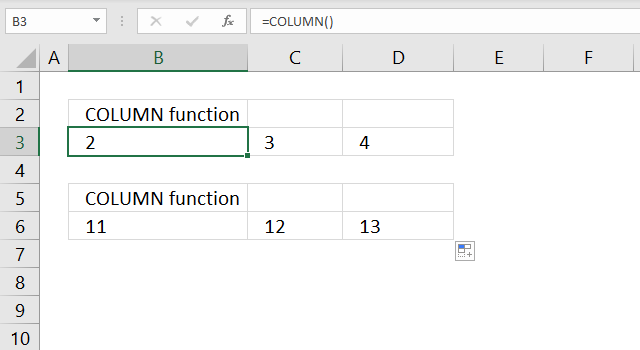The COLUMN function accepts no arguments at all, this will return the column number of the cell that the function is entered in.

Formula in cell B3:

=COLUMN()

The formula above does not change when you copy the cell and paste it to adjacent cells, however, the output is different. Cell C3 is the third column and therefore 3 is returned.

You can also use a cell reference to any other column or columns, see the formula below.

Formula in cell B6:

=COLUMN(K1)

This formula returns the column number of cell K1 which is the argument used in this example. The cell reference is a relative cell reference meaning it changes when you copy the cell and pastes it to adjacent cells, see cell range C6:D6 in the image above.

The formula below returns an array of numbers 11, 12, and 13. They correspond to the cells in cell range K1:M1 and represent their column numbers.

Formula:

=COLUMN(K1:M1)

You need to enter this formula as an array formula in order to see all numbers in the array unless you are using Excel 365. Note that the array is a horizontal array with the same size as there are cells in the supplied cell range.

## 6. COLUMN function with a cell ref to a cell range?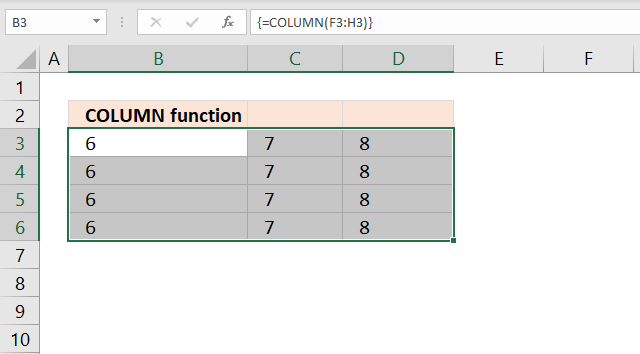The COLUMN function repeats the array of numbers if entered in a cell range.

Array formula in cell B3:

=COLUMN(F3:H3)

Note, the formula is entered as a regular formula if your Excel version is 365.

## 7. Create a horizontal sequence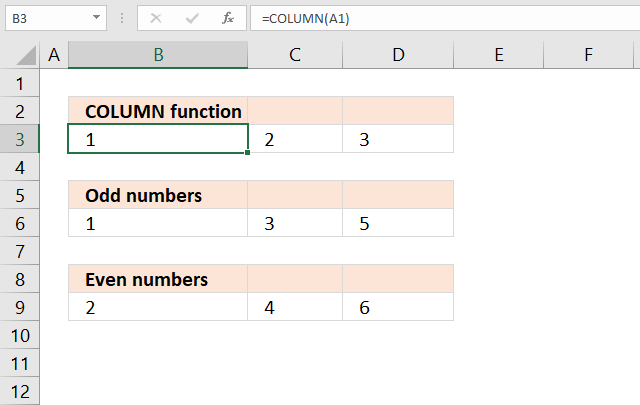The COLUMN function can create a sequence of numbers that can be useful both in formulas and on a worksheet.

Formula in cell B3:

=COLUMN(A1)

The formula above creates a horizontal sequence from 1 to n or as far as you copy the formula.

Formula in cell B6:

=(COLUMN(A1)-1)*2+1

The formula in cell B6 creates a horizontal sequence containing odd numbers from 1 to n. Copy cell B6 and paste to adjacent cells on the same row as far as needed.

Formula in cell B9:

=COLUMN(A1)*2

The formula in cell B9 creates a horizontal sequence containing odd numbers from 1 to n.

Note, relative cell references and the COLUMN function are sensitive to inserting rows and columns. The formula will break if you insert a column or row before the formula.

Use the COLUMNS function with a cell reference containing both an absolute and relative cell reference to solve this problem.

=COLUMNS(\$A\$1:A1)

## 8. COLUMN function and SMALL function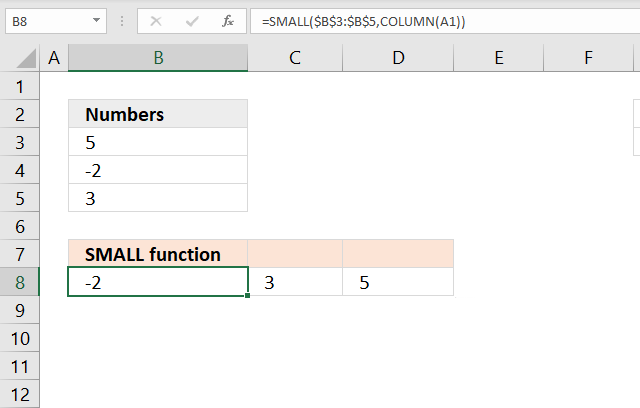The COLUMN function combined with the SMALL function can extract and sort numbers from a cell range or array.

Formula in cell B8:

=SMALL(\$B\$3:\$B\$5, COLUMN(A1))

This is a regular formula.

### Explaining formula in cell B8

#### Step 1 - Calculate sequence number

COLUMN(A1) returns 1.

#### Step 2 - Evaluate SMALL function

The SMALL function returns the k-th smallest value from a group of numbers. The first argument is a cell range or array that you want to find the k-th smallest number from.

The second and last argument is k which is a number from 1 up to the number of values you have in the first argument.

SMALL(arrayk)

SMALL(\$B\$3:\$B\$5, COLUMN(A1))

becomes

SMALL(\$B\$3:\$B\$5, 1)

becomes

SMALL({5; -2; 3}, {1})

and returns -2.

## 9. How to use the COLUMN function in VBA?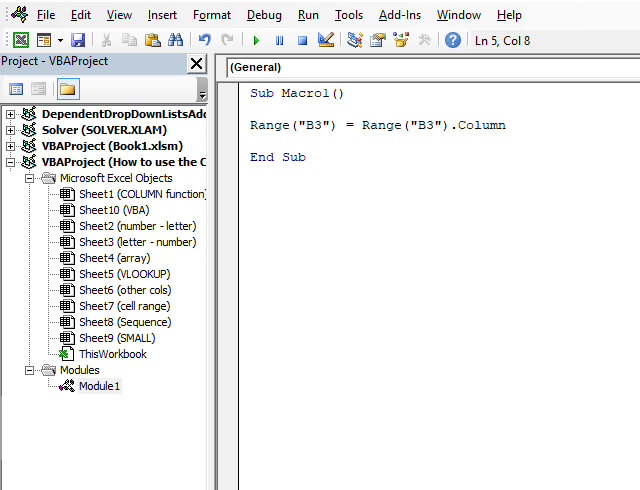The COLUMN function does not exist in the WorksheetFunction object. You can use the RANGE.COLUMN property to achieve the same thing.

The macro below saves the column number from cell B3 to cell B3. Column B is the second column.

```Sub Macro1()

Range("B3") = Range("B3").Column

End Sub
```